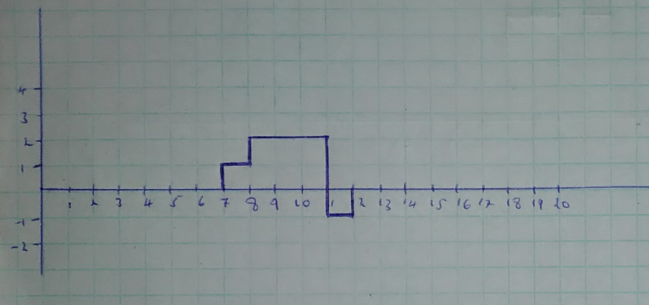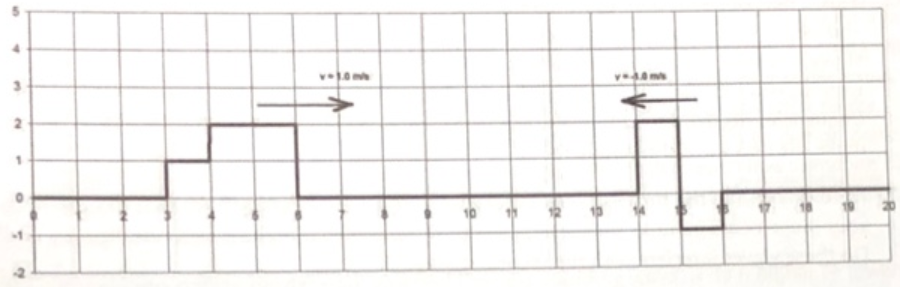# Problem: The graph shows a snapshot of two waves on a string moving towards each other at time t = 0 sec. The pulse on the left has a velocity of +1.0 m/s and the pulse on the right has a velocity of - 1.0 m/s. The horizontal axis is the position down the length of the string measured in meters and the vertical axis shows the displacement of the wave in centimeters. Draw graphs of the waves at t = 4s, t = 5s, t = 6 s and t = 9 s.

###### FREE Expert Solution

The graphs of the wave at different times can be drawn as follows:

At t = 4s93% (86 ratings)###### Problem Details

The graph shows a snapshot of two waves on a string moving towards each other at time t = 0 sec. The pulse on the left has a velocity of +1.0 m/s and the pulse on the right has a velocity of - 1.0 m/s. The horizontal axis is the position down the length of the string measured in meters and the vertical axis shows the displacement of the wave in centimeters. Draw graphs of the waves at t = 4s, t = 5s, t = 6 s and t = 9 s.